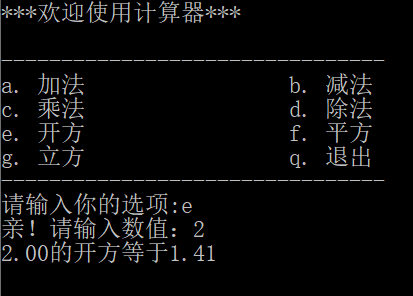C语言编程：简易计算器1个回答

``````#include<stdio.h>
#include <math.h>

{
printf("***欢迎使用计算器***\n");
printf("\n");
printf("——————————————\n");
printf("a.加法               b.减法\n");
printf("c.乘法               d.除法\n");
printf("e.开方               f.平方\n");
printf("g.立方               q.退出\n");
printf("——————————————\n");
}
int main()
{
float a, b, sum;
char ch;
printf("请输入你的选项：");
scanf("%c", &ch);
printf("亲！请输入数值：");
switch (ch)
{
case 'a':
{
scanf("%f %f", &a, &b);
sum = a + b;
printf("%.2f + %.2f = %.2f", a, b, sum);
break;
}
case 'b':

{
scanf("%f %f", &a, &b);
sum = a - b;
printf("%.2f - %.2f = %.2f", a, b, sum);
break;
}
case 'c':
{
scanf("%f %f", &a, &b);
sum = a * b;
printf("%.2f * %.2f = %.2f", a, b, sum);
break;
}
case 'd':
{
scanf("%f %f", &a, &b);
if (b == 0)
printf("除数不能为0");
else
{
sum = a / b;
printf("%.2f / %.2f = %.2f", a, b, sum);
}
break;
}
case 'e':
{
scanf("%f", &a);
sum = sqrt(a);
printf("%.2f的开方等于%.2f", a, sum);
break;
}
case 'f':
{
scanf("%f", &a);
sum = a * a;
printf("%.2f的平方等于%.2f", a, sum);
break;
}
case 'g':
{
scanf("%f", &a);
sum = a * a * a;
printf("%.2f的立方等于%.2f", a, sum);
break;
}
default:
break;
}
return 0;
}
``````